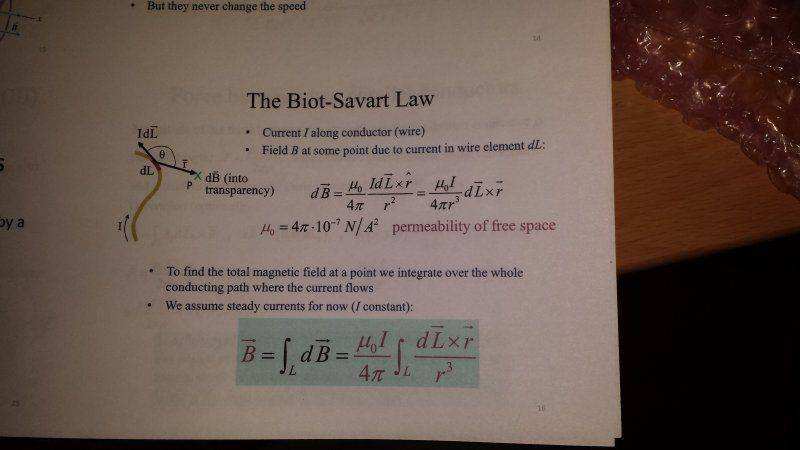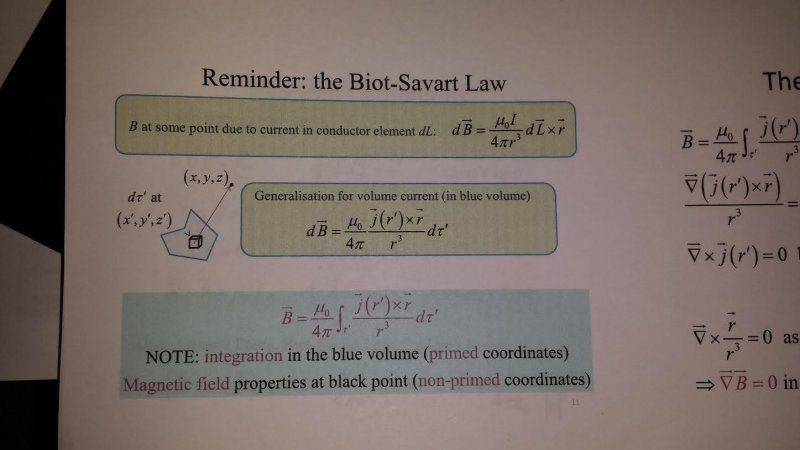# Biot-Savart for current density in a volume

henrybrent

## Homework Statement

The Biot-Savart law for a current density j in a volume V is: $d\vec{B} = \frac{\mu_0\vec{j}\times\vec{r}}{4\pi r^3} dV$

Derive the formula for the magnetic field created by a single point-like particle carrying charge $q$ moving with velocity $\vec{v}$. Explain and justify all important steps.

## Homework Equations

$d\vec{B} = \frac{\mu_0\vec{j}\times\vec{r}}{4\pi r^3} dV$

## The Attempt at a Solution

If I integrate over the whole volume, that will give me B, but it wont be correct for a moving point charge?

$\vec{B} = \frac{\mu_0}{4\pi} \int \frac{\vec{j}\times\vec{r}}{ r^3} dV$

Mentor
Why not?
You have to think about how to get a current from a point particle with an "infinite" charge density.

henrybrent
Why not?
You have to think about how to get a current from a point particle with an "infinite" charge density.

Sorry, can you elaborate ?

Mentor
What is your distribution of j? You'll need it to evaluate your integral.

henrybrent
What is your distribution of j? You'll need it to evaluate your integral.
I'm not sure, but;

$\vec{B} = \frac{\mu_0}{4\pi} \int \frac{\vec{j}\times\vec{r}}{ r^3} dV$ then,
$\vec{B} = \frac{\mu_0}{4\pi} \int \frac{\vec{v}\times\hat{r}}{ r^2} dq$

Am I on the right lines?

Mentor
j and v are not the same. They have completely different units, for example, and v does not depend on the position. Also, your equation is now independent of q, which clearly shows something is wrong.

henrybrent
j and v are not the same. They have completely different units, for example, and v does not depend on the position. Also, your equation is now independent of q, which clearly shows something is wrong.

These are all my lecture notes on the Biot-Savart Law.That's literally all I have to go on, my lecturer barely went over it at all. I have some textbooks as well, but they just provide me with what I wrote earlier, which you said was wrong?

Mentor
That's literally all I have to go on, my lecturer barely went over it at all. I have some textbooks as well, but they just provide me with what I wrote earlier, which you said was wrong?
I don't see any j=v there.

Let's start with an easier example: you have a ball of total charge Q with radius R and a uniform charge density. Its center is currently at the origin of the coordinate system, and it is moving in x-direction with a speed v. What is the current density for every point in space?

henrybrent
I don't see any j=v there.

Let's start with an easier example: you have a ball of total charge Q with radius R and a uniform charge density. Its center is currently at the origin of the coordinate system, and it is moving in x-direction with a speed v. What is the current density for every point in space?

I/A ?

Last edited:
Mentor
No, whatever I/A is supposed to mean.

henrybrent
No, whatever I/A is supposed to mean.
Sigh, then I don't know. I really don't. I know you can't tell me either.

Those pictures I have included are everything I have been taught (taught is an exceptionally strong word to use) regarding Biot-Savart law, our lecturer did not even derive them as he said you can't 'technically' derive them.

I have said this in the many other threads I've had to create, I'm not a physics student, I just take a module in Electromagnetism. I am not (necessarily..) asking to be spoon fed, but it's safe assume I know next to nothing regarding the Biot-Savart Law.

This forum is just another resource I've had to find because my textbooks assume the reader already has a sound base in Physics/maths - which I do not. Alas, I cannot keep using this an excuse.

Mentor
The current subproblem has nothing to do with Biot-Savart.

What do you know about current densities?
Can you find something like "current density is ... per ... and ..."?